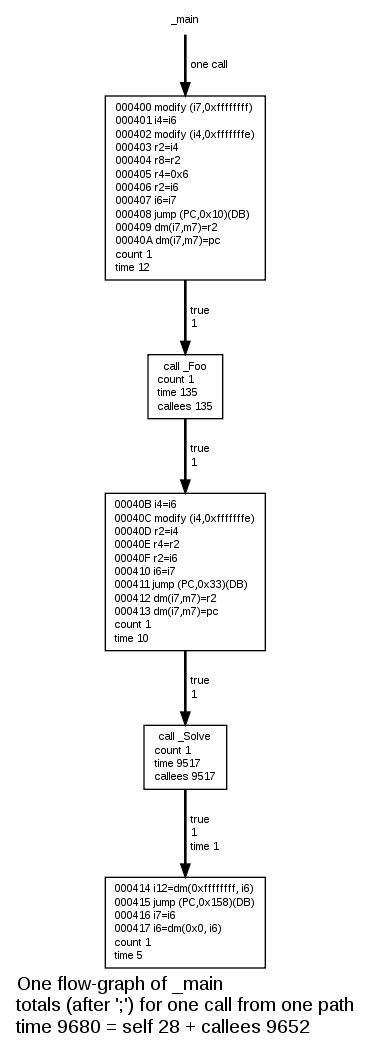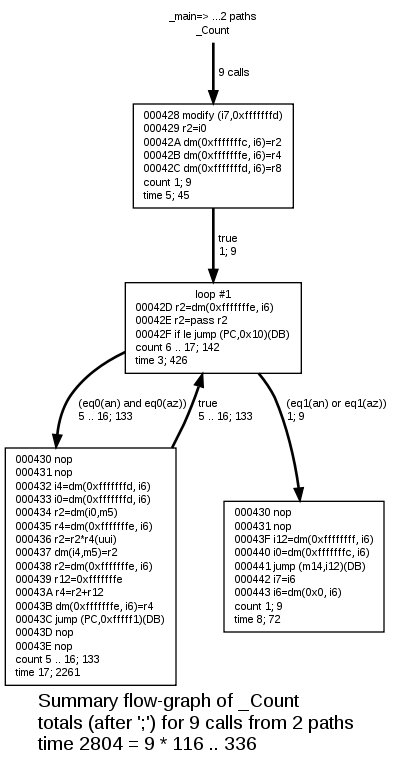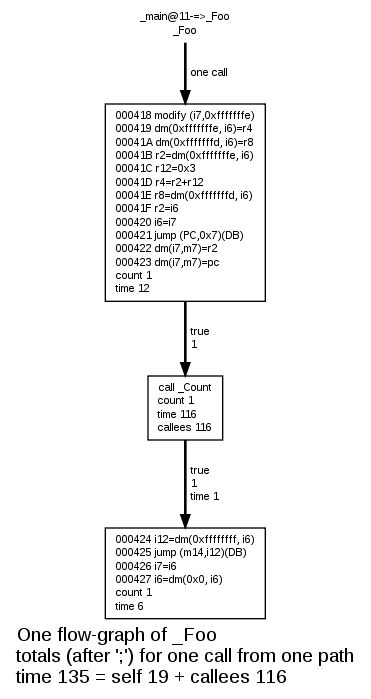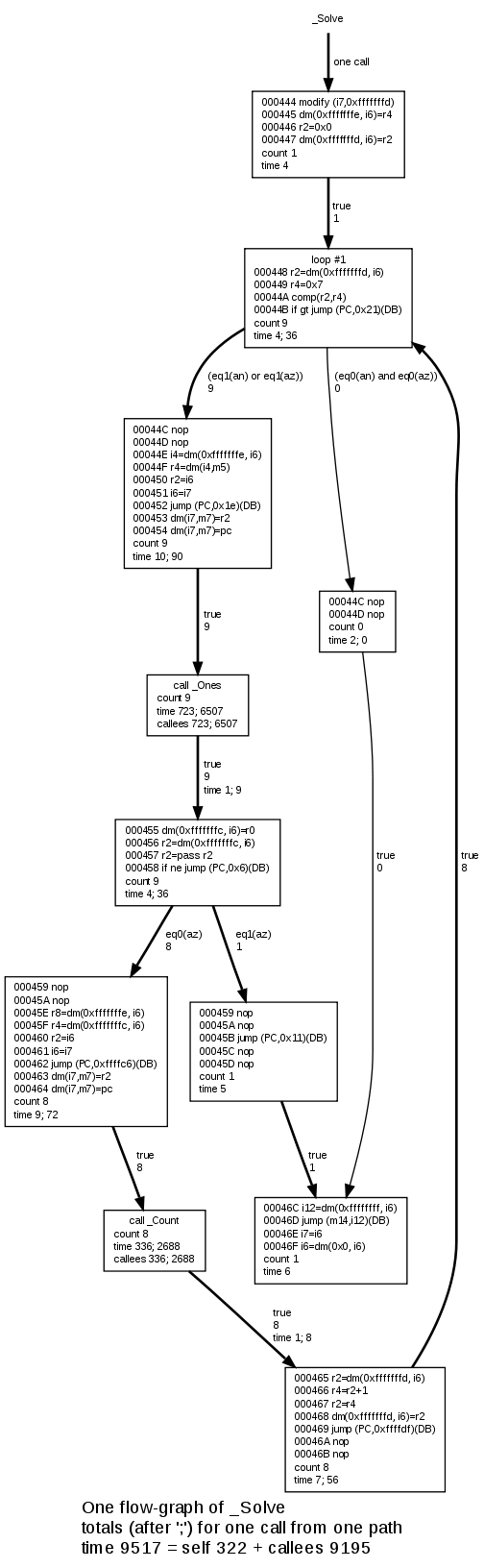## Flow-graphs from Bound-T analysis of the ADSP-21020 example

### Flow graph of main

Bound-T can draw control-flow graphs (or flow graphs, for short) of the analysed subprograms. The image on the right shows the simple flow graph of the main subprogram. It begins with the subprogram name at the top and an arrow from the name, to the box that represents the entry point of the subprogram.

Most boxes in a flow-graph diagram represents basic blocks of code. A basic block is a sequence of instructions that is entered only at the first instruction and left only at the last instruction. Depending on command-line options Bound-T can label (fill in) the box with various data about the basic block. In this example we have used the option "-draw decode" to include the disassembled instructions and their addresses, and the option "-draw no_line" to omit the name of the source-code file and the numbers of the source lines that give rise to these instructions. The last two text lines in each box show the number of times this basic block is executed in the worst-case scenario ("count") and the WCET bound ("time").

The other boxes in a flow-graph diagram represent the execution of other sub­programs due to calls from the present subprogram. These boxes are labelled with "call <callee name>" followed by the execution "count" and the execution "time" bound. The last line in the box for a callee shows the part of the time that is spent in the callee (which currently is all of the "time" for this box; in a future version of Bound-T there will be an option to compress the flow-graphs by combining these call boxes with the basic-block box that contains the call instruction, and then this "callees" label will be significant).

The arrows show the flow of control between the basic blocks and callees. The labels on the arrows show the logical condition of taking this arrow and how many times the arrow is executed in the worst-case scenario. Since the main subprogram has no conditional branches all the conditions are "true", and all blocks and arrows in its flow graph are executed once.

A box that has no arrows leaving it represents a basic block that ends by returning from the subprogram. In the main function the bottom block in the graph at right is a return block.

### One context, or summary total of all contexts

A flow-graph drawing can represent one execution context of the subprogram, or the total of all execution contexts of this subprogram. To be precise, in the first case the flow graph shows the sub­program's execution bounds for one context, while in the second case it shows the sum total of all calls to this subprogram, in all contexts, that are included in the worst-case scenario. The flow graph of main shows one execution context of main (and this is the only execution context since main is the root subprogram of this example analysis).

A "total" flow-graph drawing differs from a one-context drawing only when the subprogram has context-specific execution bounds in the analysis. In this example program this happens only for the subprogram Count, which has different execution bounds when it is called from subprogram Foo (when Count takes at most 116 cycles) and when it is called from subprogram Solve (when Count takes at most 336 cycles). This is illustrated in the context-specific form of the call-graph drawing.### Flow graph of Count

 The flow graph drawing on the right shows a summary of the nine calls of the Count subprogram including both execution contexts. This subprogram is more complex than the main subprogram. This subprogram contains conditional branches that form a loop, and as you can see the box at the head of the loop is labeled with "loop #1" where "1" is the number of of the loop (within this subprogram). In a summary flow-graph drawing, the execution-count and execution-time labels in boxes and arrows show first the value (or value range) for one call of the subprogram, and then after a semicolon comes the total (summary) value for all calls of the subprogram. For example, the loop-head block is executed between 6 and 17 times in each of the ten calls to Count, giving a total of 142 executions when the nine calls are summed. Each execution of this block takes 3 cycles, which totals to 426 cycles for the nine calls of Count. Note that Bound-T has not actually executed the program, but has deduced that the loop can repeat at most by these numbers, which for Count depend on the parameter values and therefore vary. Two arrows leave the loop-head block, as a result of the conditional jump instruction "if le jump..." in this block. The arrows are labeled with their logical conditions. Thus the arrow from the loop head to the return block is taken if register r2 is less or equal to zero; the other arrow is taken if r2 is greater than zero and leads to a large block that forms the body of the loop. The arrow back from this block to the loop head is unconditional and is thus labeled with the condition "true". You may wonder why the two blocks that can follow the loop-head block both start with the same instructions: the two "nop" instructions at addresses 000430 and 000431. This results from the fact that the "if le jump" instruction at the end of the loop-head block executes a delayed branch which means that the two instructions immediately after the "if le jump" are always executed, whether the jump condition is true or false, and only then does the branch "take effect" and choose between the instructions at 00043F (branch taken) or 000432 (branch not taken). Bound-T models this delayed branch by splitting the control flow immediately after the "if le jump" but putting the "delay slot" instructions (the two "nop"s) in both descendant blocks. The WCET bound given in the caption under the drawing also shows the total or summary bound including all nine calls (2804 cycles), and the range of WCET bounds for each call (from 116 to 336 cycles).### Flow-graph of Ones

The one-context form of a flow-graph drawing also shows total execution counts and times if the context in question is called more than once in the worst-case scenario. This happens here for example with the subprogram Ones.

The subprogram Ones has context-inde­pen­dent execution bounds. The flow-graph drawing at the right shows these bounds. However, the subprogram is called a total of nine times in the worst-case scenario, as indicated in the label of the entry arrow and also in the caption at the bottom. (The reason why Bound-T here over-estimates the number of calls, which is really at most eight, is explained in the main text.)

The "count" and "time" in the box and arrow labels give the value for one execution before the semicolon and for all eight executions after the semicolon.

This flow-graph illustrates a feature of the flow-graph drawings that did not appear in the earlier examples: two arrows are drawn with a thinner line than the other arrows. There are the arrows that enter and leave the basic block at address 00047E that contains only two "nop" instructions. These arrows are drawn with a thin line because they are not executed at all in the worst-case execution scenario; this is also shown by their label "0", for zero executions. In other words, this basic block is always passed over. The reason is that the alternative path through the block immediately to the left of the passed-over block takes longer, 6 cycles compared to 2 cycles, and is therefore taken as the worst case.

### Arrow styles

Bound-T uses three different line styles for arrows in flow graphs:

• bold style for arrows that are executed in the worst-case scenario.
• thin style for arrows that are not executed in the worst-case scenario, because there are other execution paths that take more time.
• dotted style for arrows that can never be executed because their conditions are false (in the present context).

This example program has no examples of the third kind of arrow, which is also called an infeasible path.### Flow-graphs of Foo and Count

The flow-graphs of Foo and Count are shown below. The former is simple (no loops, no conditionals), the latter has a loop.### Flow-graph of Solve

The flow-graph of Solve is the most complex one in this example, because of loops and conditional statements.